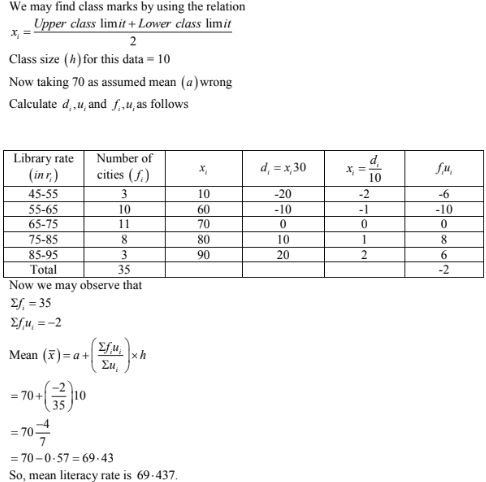# RD Sharma Solutions Chapter 7 Statistics Exercise 7.3 Class 10 Maths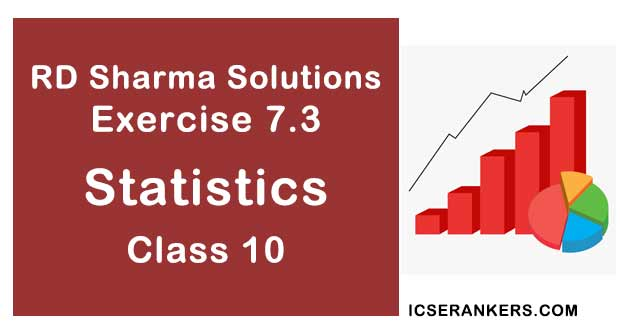Chapter Name RD Sharma Chapter 7 Statistics Book Name RD Sharma Mathematics for Class 10 Other Exercises Exercise 7.1Exercise 7.2Exercise 7.4Exercise 7.5Exercise 7.6 Related Study NCERT Solutions for Class 10 Maths

### Exercise 7.3 Solutions

1. The following table gives the distribution of total household expenditure(in rupees) of manual workers in a city.

 Expenditure (in rupees)(x) Frequency (fi) Expenditure (in rupees)(xi) Frequency(fi) 100 – 150 24 300 – 350 30 150 – 200 40 350- 400 22 200 – 250 33 400 – 450 16 250 – 300 28 450 -500 7

Find the average expenditure (in rupees) per household.

Solution

Let the assumed mean (A) = 275.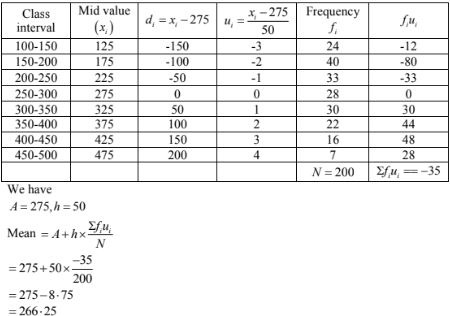2. A survey was conducted by a group of students as a part of their environment awareness program, in which they collected the following data regarding the number of plants in 20 houses in a locality. Find the mean number of plants per house.
 Number of plants: 0 – 2 2 – 4 4 – 6 6 – 8 8 – 10 10 – 12 12 – 14 Number of houses : 1 2 1 5 6 2 3

Which method did you use for finding the mean, and why ?

Solution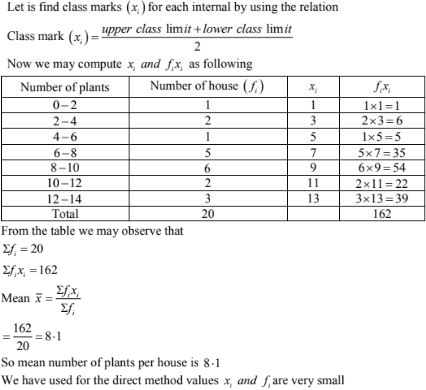3. Consider the following distribution of daily wages of 50 workers of a factory
 Daily wages(in Rs.) 100 – 120 120 – 140 140 – 160 160 – 180 180 – 200 Number of workers : 12 14 8 6 10

Find the mean dialy wages of the workers of the factory by using an appropriate method.

Solution

Let the assume mean (A) = 150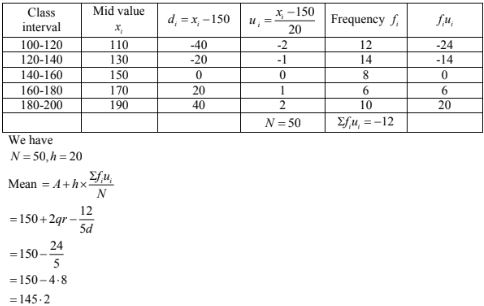4. Thirty women were examined in a hospital by a doctor and the number of heart beats per minute recorded and summarized as follows. Find the mean heart beats per minute for these women, choosing a suitable method.
 Number of heat 65 – 68 68 – 71 71 – 74 74 – 77 77 – 80 80 – 83 83 – 86 Number of women: 2 4 3 8 7 4 2

Solution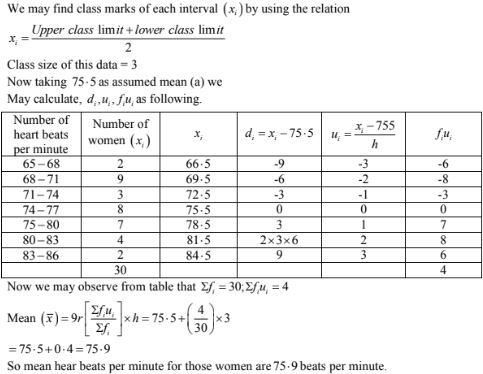5. Find the mean of each of the following frequency distributions: (5 – 14 )
 Class interval : 0 – 6 6 – 12 12 – 18 18 – 24 24 – 30 Frequency: 6 8 10 9 7

Solution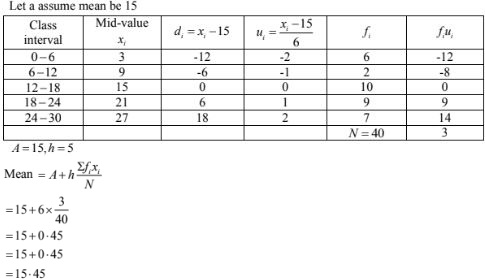6.

 Class interval: 50 – 70 70 – 90 90 – 110 110 – 130 130 – 150 150 – 170 Frequency: 18 12 13 27 8 22

Solution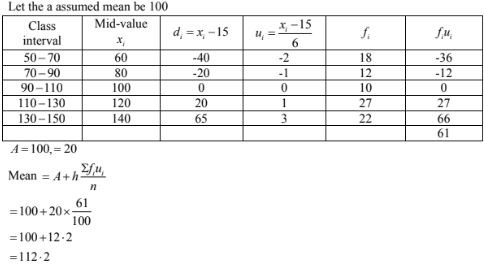7.

 Class interval: 0 – 8 8 – 16 16 – 24 24 – 32 32 – 40 Frequency: 6 7 10 8 9

Solution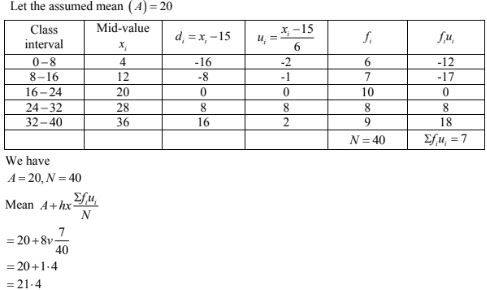8.

 Class interval: 0 – 6 6 – 12 12 – 18 18 – 24 24 – 30 Frequency : 7 5 10 12 6

Solution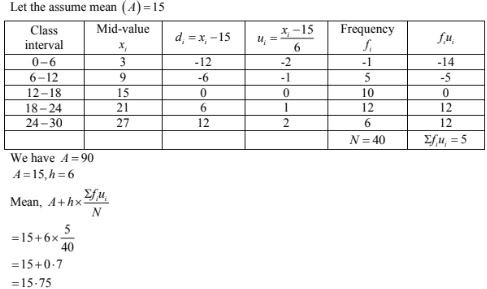9.

 Class interval : 0 – 10 10 – 20 20 – 30 30 – 40 40 – 50 Frequency: 9 12 15 10 14

Solution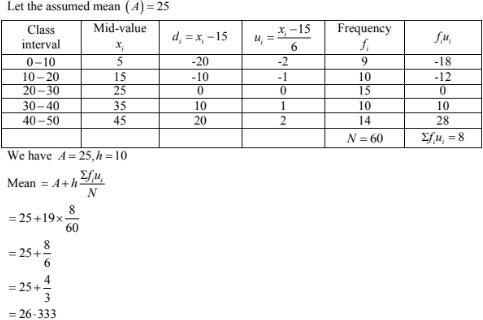10.

 Class interval : 0 – 8 8 – 16 16 – 24 24 – 32 32 – 40 Frequency: 5 9 10 8 8

Solution11.

 Class interval: 0 – 8 8 – 16 16 – 24 24 – 32 32 – 40 Frequency: 5 6 4 3 2

Solution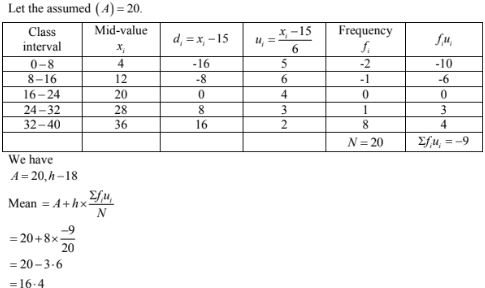12.

 Class interval: 10 – 30 30 – 50 50 – 70 70 – 90 90 – 110 110 – 130 Frequency: 5 8 12 20 3 2

Solution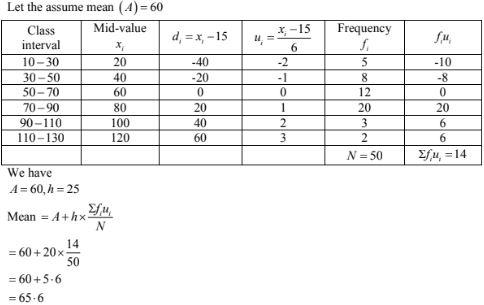13.

 Class interval: 25 – 35 35 – 45 45 – 55 55 – 65 65 – 75 Frequency: 6 10 8 12 4

Solution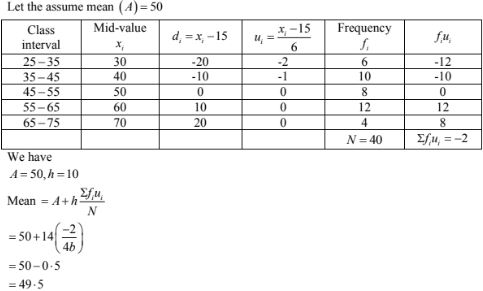14.

 Classes: 25 – 29 30 – 34 35 – 39 40 – 44 45 – 49 50 – 54 55 – 59 Frequency: 14 22 16 6 5 3 4

Solution15. For the following distribution, calculate mean using all suitable methods:
 Size of item: 1 -4 4 – 9 9 – 16 16 – 27 Frequency: 6 12 26 20

Solution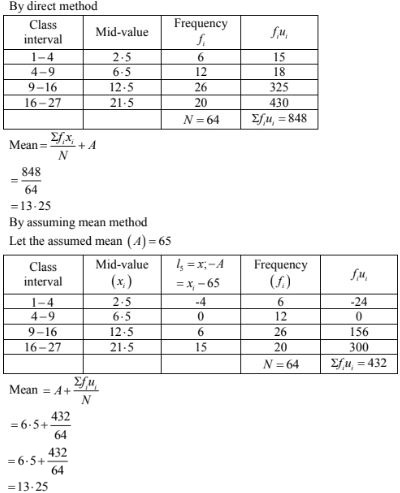16. The weekly observations on cost of living index in a certain city for the year 2004 – 2005 are given below. Compute the weekly cost of living index.
 Cost of living index Number of Students Cost of living index Number of Students 1400 – 1500 5 1700 – 1800 9 1500 – 1600 10 1800 – 1900 6 1600 - 1700 20 1900 – 2000 2

Solution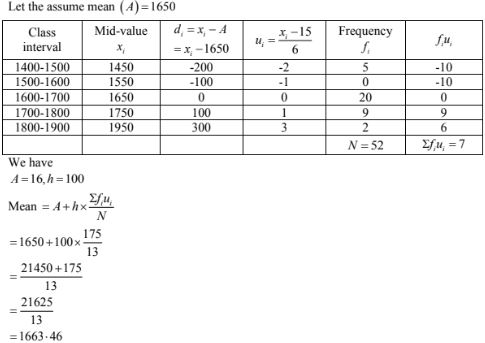17. The following table shows the marks scored by 140 students in an examination of a certain paper:
 Marks: 0 – 10 10 – 20 20 – 30 30 – 40 40 – 50 Number of students : 20 24 40 36 20

Calculate the average marks by using all the three methods: direct method, assumed mean deviation and shortcut method.

Solution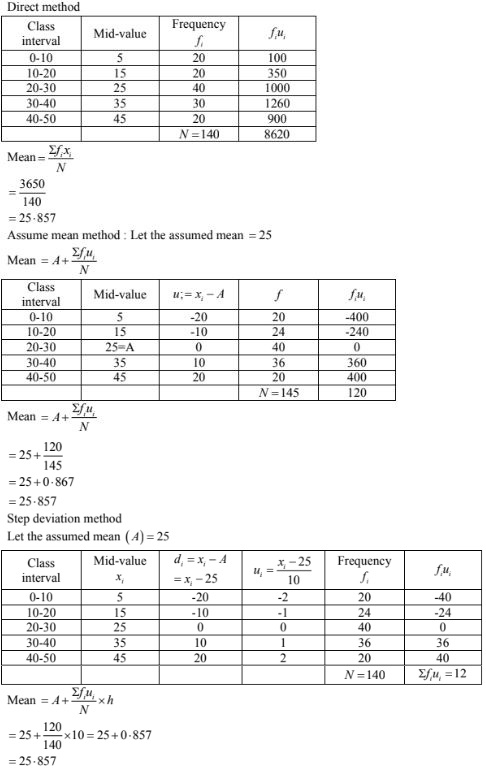18. The mean of the following frequency distribution is 62.8 and the sum of all the frequencies is 50. Compute the missing frequency f1 and f2 .
 Class: 0 – 20 20 – 40 40 – 60 60 – 80 80 – 100 100 – 120 Frequency: 5 F1 10 F2 7 8

Solution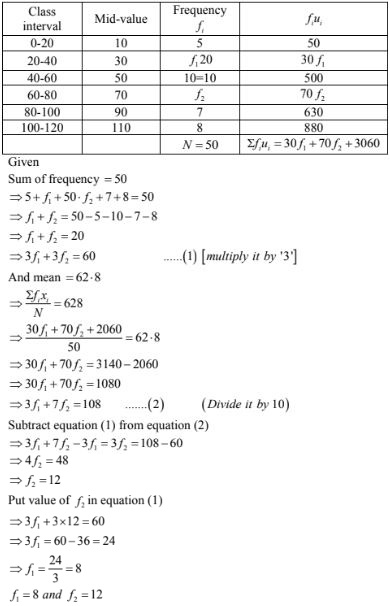19. The following distribution shows the daily pocket allowance given to the children of a multistorey building. The average pocket allowance is Rs 18.00. Find out the missing frequency.
 Class interval: 11 – 13 13 – 15 15 – 17 17 – 19 19 – 21 21 – 23 23 – 25 Frequency: 7 6 9 13 - 5 4

Solution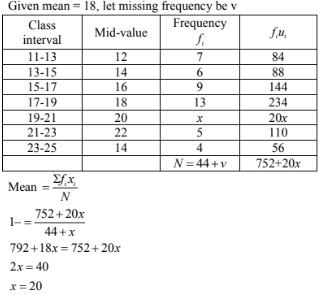20. If the mean of the following distribution is 27, find the value of p.
 Class : 0 – 10 10 – 20 20 – 30 30 – 40 40 – 50 Frequency: 8 P 12 13 10

Solution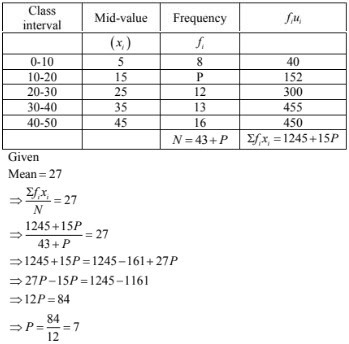21. In a retail market, fruit vendors were selling mangoes kep in packing boxes. These boxes contained varying number of mangoes. The following was the distribuion of mangoes according to the number of boxes.
 Number of mangoes: 50 – 52 53 – 55 56 – 58 59 -61 62 -64 Number of boxes: 15 110 135 115 25

Solution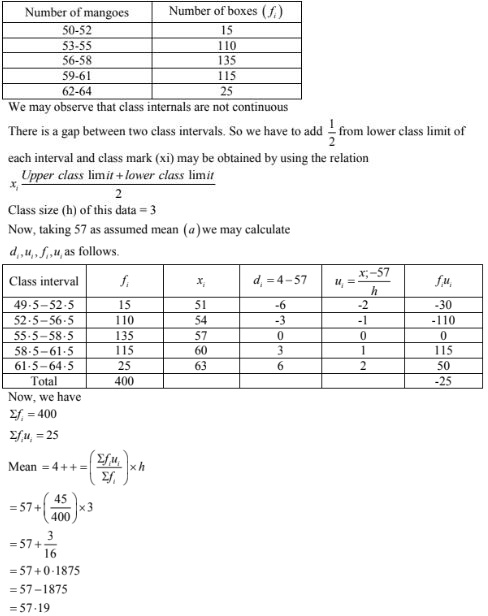22. The table below shows the daily expenditure on food of 25 household in a locality.
 Daily expenditure(in Rs): 100 – 150 150 – 200 200 – 250 250 – 300 300 – 350 Number of households: 4 5 12 2 2

Find the mean daily expenditure on food by a suitable method.

Solution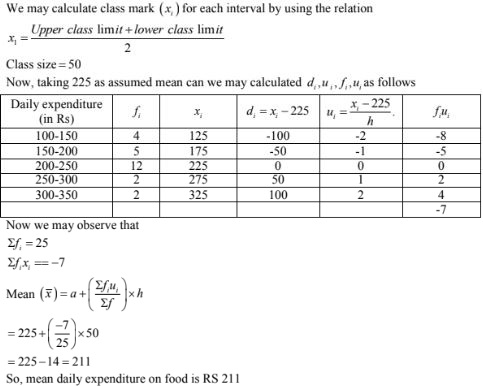23. To find out the concentration of SO2 in the air (in parts per million, i.e., ppm), the data was collected for 30 localities in a certain city and is presented below:
 Concentration fo SO2 (in ppm) Frequency 0.00 – 0.04 4 0.04 – 0.08 9 0.08 – 0.12 9 0.12 – 0.16 2 0.16 – 0.20 4 0.20 – 0.24 2

Find the mean concentration of SO2 in the air.

Solution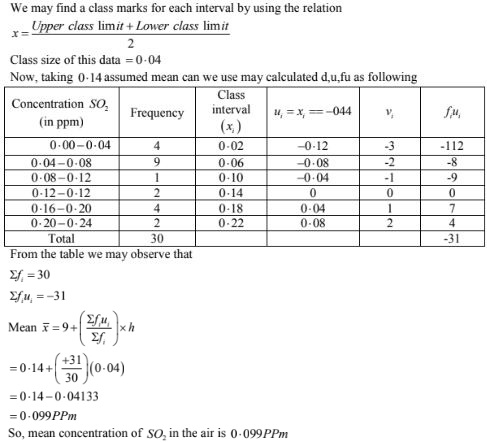24. A class teacher has the following absentee record of 40 students of a class for the whole term. Find the mean number of days a student was absent.
 Number of days: 0 – 6 6 – 10 10 – 14 14 – 20 20 – 28 28 – 38 38 – 40 Number of students: 11 10 7 4 4 3 1

Solution

We may find class mark of each interval by using the relation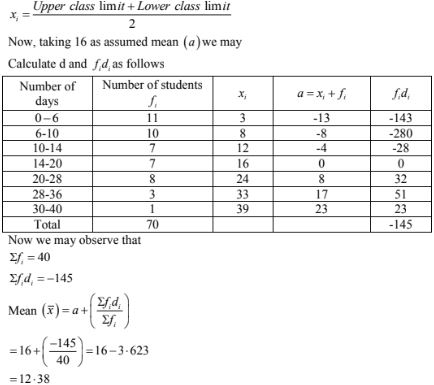So, mean number of days is 12, 38 days for which student was absent.

25. The following table gives the literacy rate(in percentage) of 35 cities. Find the mean literacy rate.
 Literacy rate(in %): 45 – 55 55 – 65 65 – 75 75 – 85 85 – 95 Number of cities: 3 10 11 8 3

Solution